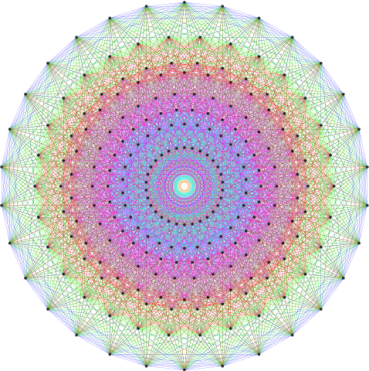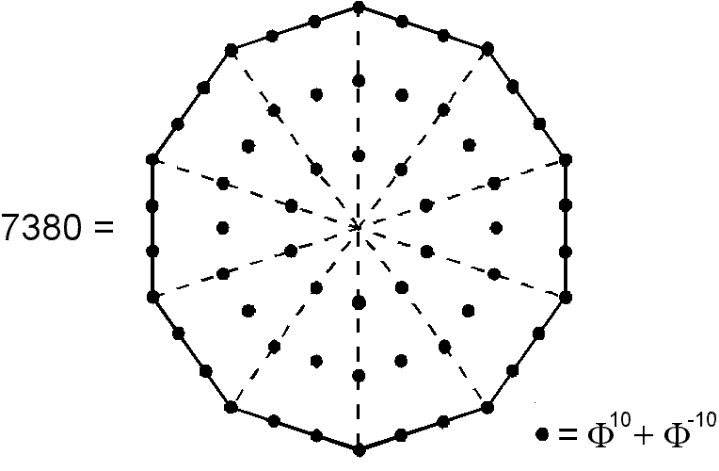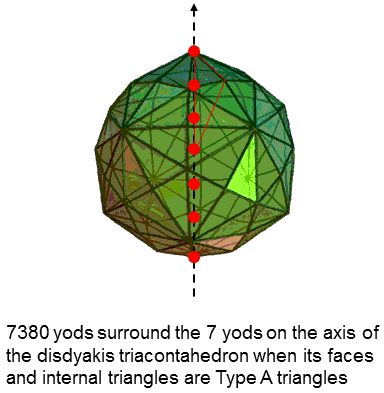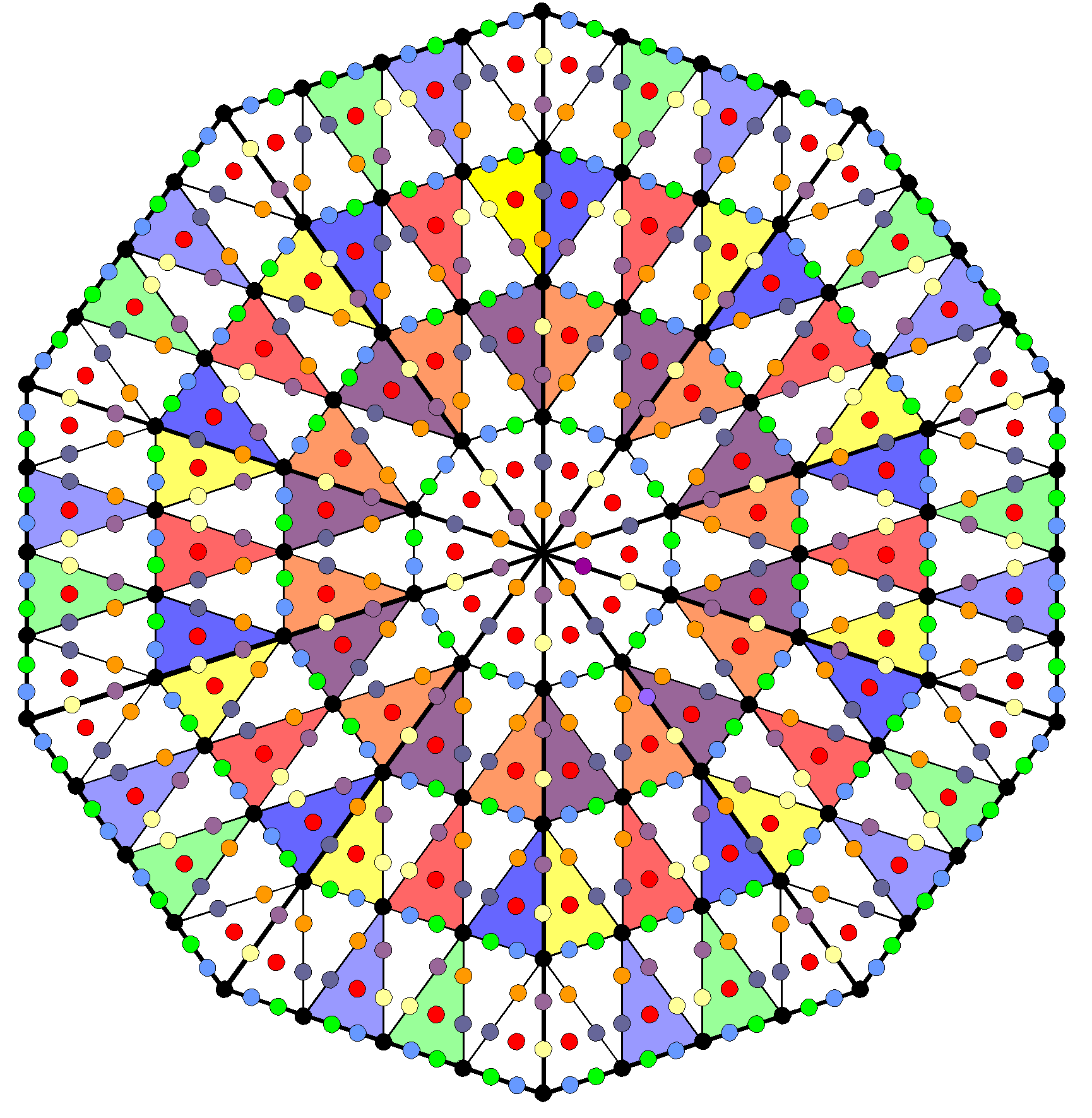Home

 << Previous    1...   16  17    19  20  ...24    Next >>

18. The disdyakis triacontahedron embodies the number of edges of the 421 polytopeThe 421 polytope has 6720 edges. (A) 6720 yods other than vertices are needed to construct the faces & interior of the disdyakis triacontahedron from Type A and Type B triangles; (B) 6720 yods other than new corners are needed to construct the faces & interior of the disdyakis triacontahedron from Type A triangles.

There are two possible ways in which the faces and interior of the disdyakis triacontahedron can be constructed from Type A and Type B triangles in order for it to embody the number 6720:

A Type A triangle contains 19 yods. Nine yods line its sides and 10 yods lie inside its boundary (one corner & nine hexagonal yods in three tetractyses). A Type B triangle contains 46 yods. Nine line its sides and 37 are internal (four corners and 33 hexagonal yods in nine tetractyses).

Case A. Type B triangular faces & Type A internal triangles extended by edges

Faces: The 120 Type B triangles have (120×9=1080) tetractyses with (120×4=480) internal corners and (120×33=3960) internal hexagonal yods. They have 60 external corners surrounding an axis passing through two diametrically opposite vertices.
Edges: (180×2=360) hexagonal yods line 180 edges.
Interior: The 180 Type A internal triangles have 180 internal corners and (180×9=1620) hexagonal yods. Their sides have (60×2=120) hexagonal yods.

The number of corners = 60 + 480 + 180 = 720. The number of hexagonal yods = 3960 + 360 + 1620 + 120 = 6060. The total number of yods surrounding the axis = 720 + 6060 = 6780. Therefore, (6780−60=6720) yods other than vertices surround the axis.

Case B. Type A triangular faces & Type A internal triangles extended by sides of sectors of faces

Faces: The 120 Type A triangles have (120×3=360) tetractyses with 120 internal corners and (120×9=1080) internal hexagonal yods. They have 60 external corners surrounding an axis passing through two diametrically opposite vertices.
Edges: (180×2=360) hexagonal yods line 180 edges.
Interior: The 180 edges extend 180 Type A triangles with 180 internal corners of (180×3=540) tetractyses and (180×9=1620) hexagonal yods. Their internal sides have (60×2=120) hexagonal yods. The (120×3=360) sides of tetractyses in the faces extend 360 Type A triangles with 360 internal corners of (360×3=1080) tetractyses and (360×9=3240) hexagonal yods. Their sides have (120×2=240) hexagonal yods as well as the 120 hexagonal yods generated by sides joining the centre of the polyhedron to vertices.

The number of corners = 60 + 120 + 180 + 360 = 720. The number of hexagonal yods = 1080 + 360 + 1620 + 120 + 3240 + 240 = 6660. The total number of yods surrounding the axis = 720 + 6660 = 7380. Therefore, (6660+60=6720) yods other than new corners of tetractyses are needed to construct the disdyakis triacontahedron. This is how the disdyakis triacontahedron embodies the holistic parameter 6720 that manifests in the 421 polytope as its 6720 edges.

It can be argued that Case A is illegitimate because it treats the exterior and interior triangles inconsistently, the faces being regarded as Type B triangles and interior triangles being treated as Type A. It would be more correct to say that such an analysis would be incomplete (and, therefore, misleading on its own) because it would be describing part of a holistic system constructed solely from Type B triangles. In Case B, all faces and interior triangles are of the same type, and so it is mathematically complete. For this reason, discussion will focus on this case alone.

• The number of corners surround the axis = 720 = 6!. This is a parameter of holistic systems, being, for example, the number of hexagonal yods in the faces of the five Platonic solids (see table here) and the number of yods surrounding the centres of the seven Type B polygons of the inner Tree of Life (see here). It is also the number of edges in the 600-cell (see below Table 1a on #1)), the compound of two of which generate the vertices of the 421 polytope.
• The total number of yods surrounding the axis = 7380 = 123×60. The number 123 is the 10th Lucas number (L10) after the first number L0 = 2 in the Lucas sequence:

2, 1, 3, 4, 7, 11, 18, 29, ....

where the nth Lucas number Ln = Ln-1 + Ln-2 if n>1. As Ln = Φn + (-Φ)-n, where Φ is the Golden Ratio, 123 = Φ10 + Φ-10. Assigning the sum of the tenth power of Φ and the tenth power of its reciprocal to the 60 yods surrounding the centre of a Type A decagon (symbol of the number 10) generates the number of yods needed to construct the disdyakis triacontahedron (observe that this is the number of yods in the polyhedron that surround its axis, which comprises the seven yods lining the two vertical sides shared by those triangles that are formed by the North & South poles of the polyhedron, their nearest neighbour vertices & its centre):This demonstrates in a remarkable way how the Decad determines both arithmetically and geometrically the yod population of this polyhedral version of the Tree of Life. It also determines the number (720) of corners of all the tetractyses making up the disdyakis triacontahedron because this is the number of yods in a decagon with 2nd-order tetractyses as sectors:• The number of hexagonal yods = 6660 = 10×666 = 10×(1+2+3+...+36), where 36 is the number of ELOHA, the Godname of Geburah, which is the sixth Sephirah of Construction, counting from Malkuth. 10×36 (=360) is the number of hexagonal yods lining the 180 edges of the disdyakis triacontahedron.
• The number of corners generated by the construction of the polyhedron from Type A triangles = 720 − 60 = 660 = 66×10, where 66 is the 65th integer after 1. This is how ADONAI, the Godname of Malkuth with number value 65, prescribes the number 6720, which is the difference between 7380 (total number of yods) and 660. The number of edges of the 421 polytope is identical to the number of yods other than new corners of tetractyses that are needed to construct the disdyakis triacontahedron from Type A triangles.
• The number of tetractyses = 360 + 540 + 1080 = 1980. Each has a hexagonal yod at its centre. The number of hexagonal yods lining their sides = 6660 − 1980 = 4680. Of these, 360 hexagonal yods line the 180 edges, leaving (4680−360=4320) hexagonal yods that line 2160 (=216×10) sides of tetractyses other than edges. 216 is the number of Geburah. Here is a remarkable conjunction of the number values of both a Sephirah and its Godname in the same context! Indeed, the number of hexagonal yods other than those lining the edges = 6660 − 360 = 6300 = 630×10, where 630 is the number value of Seraphim, the Order of Angels assigned to Geburah. We now see that a chance-defying, triple conjunction of gematria number values for the same Sephirah exists in the same context! How could any sceptic reasonably dismiss this as coincidence?
• As two hexagonal yods lie on each side of a tetractys, the 1980 tetractyses have 720 corners with (4680/2=2340) sides. Constructed from them, the disdyakis triacontahedron is composed of (720+2340+1980=5040) points, lines & triangles. As 5040 = 7!, this is the number of permutations of seven objects. They comprise 720 corners, where 720 (= 6!) is the number of permutations of six objects. The number of lines & triangles = 7! − 6!. It is hard to believe that these simple properties could be coincidental and not a manifestation of a universal, mathematical design that is associated with an object possessing sacred geometry (as confirmed in many places in this website, the disdyakis triacontahedron is the polyhedral version of the Tree of Life)! The number of lines = 2340 and the number of lines & triangles = 4320. Notice the reversal of the integers 2, 3 & 4. The number of points & lines = 3060, so that each half of the disdyakis triacontahedron is composed of 1530 (=153×10) points & lines. This shows how ELOHIM SABAOTH, the Godname of Hod with number value 153, prescribes the shape of the disdyakis triacontahedron.* The number of points & lines other than vertices & edges = 3060 − 60 − 180 = 2820 = 282×10, where 282 is the number value of Aralim, the Order of Angels assigned to Binah.

* This Godname also prescribes the inner form of the Tree of Life in an analogous way because the (7+7) enfolded polygons have 153 corners & sides.

 << Previous    1...   16  17    19  20  ...24    Next >>

Home